HOME COURSES PREVIEW REVIEW ABOUT CONTACTTOLL-FREE INFO & ORDERING: M-F: 9am-5pm (PST): (877) RAPID-10

 Quick Search: Keywords:

 Rapid Learning Member Area:Note: If you are a legacy user of chemistry24 members, please request a new login access to the premium server with your full name and old login email via vip@rapidlearningcenter.com

 Rapid Courses Catalog : Physics in 24 Hours Chemistry in 24 Hours Biology in 24 Hours Mathematics in 24 Hours

Weekly Physics Tips:
Want to become a top gun in your class? How about study less yet score high? Sign up this Physics Survival Weekly to learn how. Designed specifically for students who are taking physics, this free newsletter will show you how to survive and excel in class! Weekly topics include:
• How to Study Physics Effectively
• How to Read Physics Textbooks Easily
• How to Solve Physics Problems Systematically
• How to Score High on Physics Exams Strategically
• How to Master Physics Rapidly
Each week, you will receive study tips on the topics above and visual tutorial or study template to enhance your physics learning. Enter your name and email below to subscribe free:

 Physics Study Lounge These study sheets are for quick review on the subjects. Refer to our rapid courses for comprehensive review.     - Basic Skills in Physics     - Basic Math for Physics     - How to Solve Physics Problems     - Newton's Laws Study Guide     - ElectroMagnetism Quick Review     - Atomic Physics At-A-Glance     - Core Concepts in Nuclear Physics     - Special Relativity Overview

 Other Related Sites
 Note: For course links to launch, disable popup blockers or hold the ctrl key while clicking the link.Home » College Calculus

Parametric Equations and Polar Coordinates

 Topic Review on "Title": Definition of parametric equations: Suppose that x and y are continuous functions of a third variable t. Then x=f(t) and y=g(t) are called parametric equations for the curve represented by (x,y). Lines in polar coordinates: Let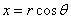and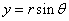then the polar equations of the lines x=a and y=b are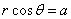and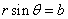for all values of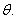Parametric form of derivatives: Suppose a smooth curve C is defined by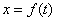and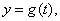then the slope of C at (x,y) is given bywithArc length of parametric curves: Suppose thatandare continuous functions on the interval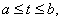then the curveand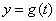has arc length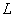is given by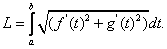Rapid Study Kit for "Title":
 Flash Movie Flash Game Flash Card Core Concept Tutorial Problem Solving Drill Review Cheat Sheet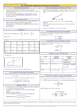"Title" Tutorial Summary : Parametric equations are presented to motivate the idea of their derivatives and how they are related to the parametric form. Examples are shown to denote the characteristics of how the derivative formulas affect the vertical tangent and the concept of a singular point. The arc length of parametric curves is shown with the use of parametric curves. Polar coordinates and their graphical interpretation are shown with the use of examples. Converting rectangular coordinates to polar coordinate is shown to motivate the idea of the arc length in polar coordinates. The polar coordinate graphs are given to discuss the idea of how you represent circles in polar coordinates. Polar coordinates are important to know in a typical Calculus course.

 Tutorial Features: Specific Tutorial Features: Several example problems with step by step illustrations of solutions Graphs showing equations in polar coordinate form and rectangular form. The different types of representation of the arc length using derivatives are presented in this tutorial. Series Features: Concept map showing inter-connections of new concepts in this tutorial and those previously introduced. Definition slides introduce terms as they are needed. Visual representation of concepts Animated examples—worked out step by step A concise summary is given at the conclusion of the tutorial.

 "Title" Topic List: `Parametric Equations Examples of parametric equations Parametric form of derivatives Arc length of parametric curvesPolar coordinates Converting Rectangular Coordinates to Polar Coordinates Area in polar coordinates Arc length in polar coordinatesGraphs in Polar Coordinates `

See all 24 lessons in college calculus, including concept tutorials, problem drills and cheat sheets:
Teach Yourself College Calculus Visually in 24 Hours